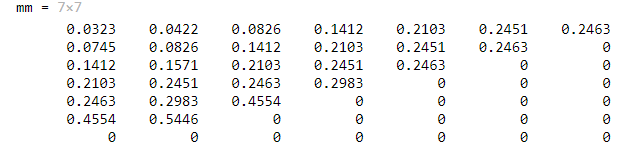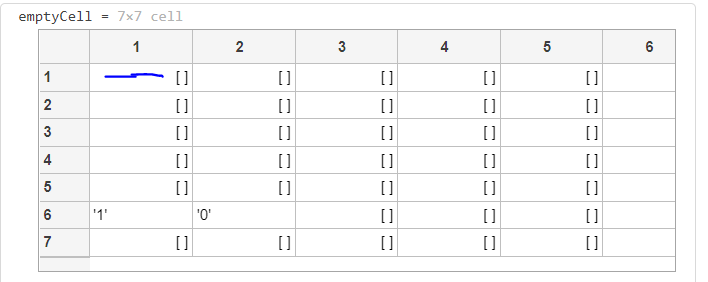# creating a character array in which each element takes multiple characters put together

7 views (last 30 days)
Hashem Rawashdeh on 22 May 2021
Commented: Rena Berman on 23 Sep 2021
I want to assign each element of an n by m matrix with a specefic code that depends on the code of other elements in the matrix
For example in the below picture I want to give the element mm(6,2) a code '0' and element mm(6,1) code'1'
the problem comes here,I want the code of the element mm(5,1) per say to be the code of mm(6,2) with an extra '1' next to its right so it becomes '01'
this is the code I have and I keep running into size problems:
x=size(mm);
xx=x(1)-1;
emptyCell = cell(7,7);
emptyCell(xx,2)={'0'};
emptyCell(xx,1)={'1'};
for i=xx-1:-1:2
temp=find((mm(i,:)==mm(i-1,2)+mm(i-1,1)));
emptyCell(i-1,2)={emptyCell(i,temp) '0'}; % here is the error
emptyCell(i-1,2)={emptyCell(i,temp) '1'}; % and here
endI want to fill the code that I assigned in emptyCell ,for example I want the blue marked cell to contain '1011',How can I approach this problem?any help would be appreciated
Rena Berman on 23 Sep 2021

per isakson on 22 May 2021
Edited: per isakson on 22 May 2021
The RHS of the two assignments in the for-loop are they correct?Don't show data as images. Seven lines can be included as code.
In this case, I think it's better to use a string array than a cell array of characters (emptyCell). The code becomes easier to read and understand.
I don't fully understand your algorithm.
Below is a code that doesn't throw errors. In the two latter iterations temp is empty. ( == and floating point numbers is not a good combination.) For some reason {horzcat(emptyCell{i,temp},'0')} doesn't throw errors in that case. I believe that {horzcat(emptyCell{i,temp},'0')} does what you intended.
%%
mm = [ 0.0323 0.0422 0.0826 0.1412 0.2103 0.2451 0.2463
0.0745 0.0826 0.1412 0.2103 0.2451 0.2463 0
0.1412 0.1571 0.2103 0.2451 0.2463 0 0
0.2103 0.2451 0.2463 0.2983 0 0 0
0.2463 0.2983 0.4554 0 0 0 0
0.4554 0.5446 0 0 0 0 0
0 0 0 0 0 0 0 ];
%%
x=size(mm);
xx=x(1)-1;
emptyCell = repmat( {''}, 7,7 );
emptyCell(xx,2)={'0'};
emptyCell(xx,1)={'1'};
for i=xx-1:-1:2
temp = find((mm(i,:)==mm(i-1,2)+mm(i-1,1)));
emptyCell(i-1,2) = {horzcat(emptyCell{i,temp},'0')};
emptyCell(i-1,1) = {horzcat(emptyCell{i,temp},'1')};
end
%%
x = size(mm);
xx = x(1)-1;
str = strings(7,7);
str(xx,2) = "0";
str(xx,1) = "1";
for jj = xx-1:-1:2
pos = find( mm(jj,:) == (mm(jj-1,2)+mm(jj-1,1)) );
if not(isempty( pos ))
str(jj-1,2) = str(jj,pos)+"0";
str(jj-1,1) = str(jj,pos)+"1";
else
fprintf('.');
end
end